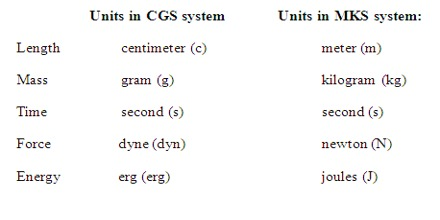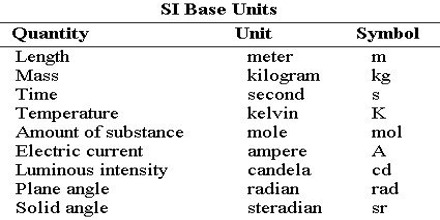# System of Units

In addition to System of Units, as per requirement of different branches of physics there is one or more necessary and appropriate standard quantity and adding its associate unit there are two more methods in use. All these methods are discussed below.

(i) Centimetre-Gramme-Second System or Metric System or French System: This method is abbreviated as C. G. S. method (or cm. gm. sec) method.

Here,

• C stands for centimeter:- the unit of length
• G stands for gramme:- the unit of mass
• S stands for second:- the unit of time

That means, in this method unit of length is centimeter, unit of mass is gramme and unit of time is second. This method is called Decimal System.(ii) Metre-Kilogramme-Second System: This method is abbreviated as M. K. S. system.

Here,

• M stands for metre:- the unit of length
• K stands for kilogramme:- the unit of mass
• S stands for second:- the unit of tune

That means, in this system tout of length is metre, the unit of mass is kilogramme and unit of time is second.

(iii) International System of Units or S. I. Units: In different countries there are different units in use. In some countries C.G.S. system, to some countries, F.P.S. system and again in some countries M.K.S system as in use. There exists inconvenience in practical field because of these variations. In order to remove this discrepancy and inconvenience scientists of different countries introduced, except the above three systems, a new system of unit in I960. This is the International System of Units or S. I. Units.

Adding some more standard quantities and then units with the existing M.K.S. system this International system has been introduced. In the following list, different quantities, their units and symbols have been mentioned. In this system there are nine quantities.